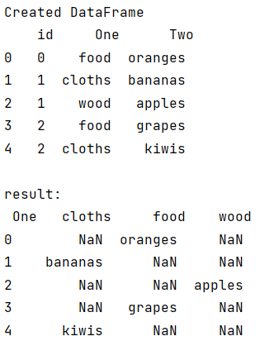# Pandas column values to columns

Given a Pandas DataFrame, we have to explode column into multiple columns.
Submitted by Pranit Sharma, on July 21, 2022

Pandas is a special tool that allows us to perform complex manipulations of data effectively and efficiently. Inside pandas, we mostly deal with a dataset in the form of DataFrame. DataFrames are 2-dimensional data structures in pandas. DataFrames consist of rows, columns, and data.

Sometimes we perform some complex operations on our DataFrame to draw some complex but useful insights from the data. In that case, we sometimes need to explode a column/series into multiple columns of a pandas Dataframe.

To explode column into multiple columns, we are going to use pandas.DataFrame.pivot() method.

## pandas.DataFrame.pivot() Method

This method is used to reshape the given DataFrame according to index and column values. It is used when we have multiple items in a column, we can reshape the DataFrame in such a way that all the multiple values fall under one single index or row, similarly we can convert these multiple values as columns.

Syntax:

```DataFrame.pivot(
index=None,
columns=None,
values=None
)
```

To work with pandas, we need to import pandas package first, below is the syntax:

```import pandas as pd
```

Let us understand with the help of an example,

## Python code for Pandas column values to columns

```# Importing pandas package
import pandas as pd

# Creating a Dictionary
d = {
'id':[0,1,1,2,2],
'One':['food','cloths','wood','food','cloths'],
'Two':['oranges','bananas','apples','grapes','kiwis']
}

# Creating a DataFrame
df = pd.DataFrame(d)

# Display DataFrame
print("Created DataFrame\n",df,"\n")

# Pivot dataframe
result = df.pivot_table(values='Two', index=df.index, columns='One', aggfunc='first')

# Display result
print("result:\n",result)
```

Output:What's New

Top Interview Coding Problems/Challenges!

Languages: » C » C++ » C++ STL » Java » Data Structure » C#.Net » Android » Kotlin » SQL
Web Technologies: » PHP » Python » JavaScript » CSS » Ajax » Node.js » Web programming/HTML
Solved programs: » C » C++ » DS » Java » C#
Aptitude que. & ans.: » C » C++ » Java » DBMS
Interview que. & ans.: » C » Embedded C » Java » SEO » HR
CS Subjects: » CS Basics » O.S. » Networks » DBMS » Embedded Systems » Cloud Computing
» Machine learning » CS Organizations » Linux » DOS
More: » Articles » Puzzles » News/Updates